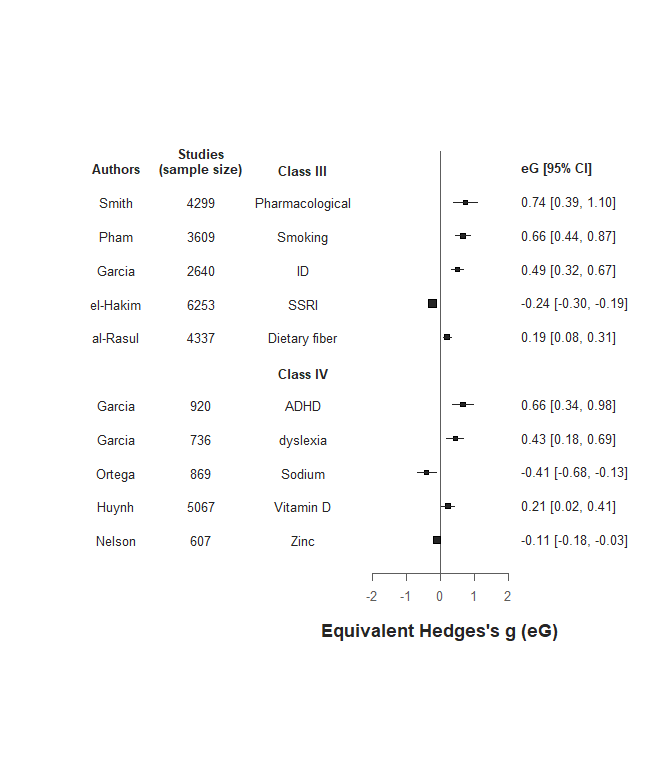# Introduction

As in any statistical analysis, data visualization is an important step to interpret the results. To do so, the metaumbrella package proposes to display the results of your umbrella review using forest plots adapted to umbrella reviews. Contrary to forest plots used in standard meta-analyses (in which the effect sizes of individual studies are plotted), the forest plots proposed in the metaumbrella package display the pooled effect sizes of the meta-analyses.

More precisely, the graphical presentation of the results generated by the forest function includes (i) the name of the factors assessed in the umbrella review (referred to as the factor column), (ii) a graphical representation of the pooled effect size of each factor using a forest plot, and (iii) the numeric values of the pooled effect size and 95% confidence interval (referred to as the value column). Numerous options are proposed to customize this basic figure.

In the examples provided below, we present how to display a simple forest plot in which some design elements are modified (such as the police or the size of the dots; Example 1), how to display a more complex forest plot in which the position of the two core columns are modified (Example 2) and how to display a forest plot in which several additional columns have been added and in which factors with specific classes are removed (Example 3).

# Example 1: simple forest plot

This example uses the dataset named df.OR distributed along with the metaumbrella package. In this example, we plot the results of the calculations of the umbrella review while no stratification of evidence has been performed.

# perform the calculations
umb <- umbrella(df.OR, verbose = FALSE)

# plot the results
forest(umb)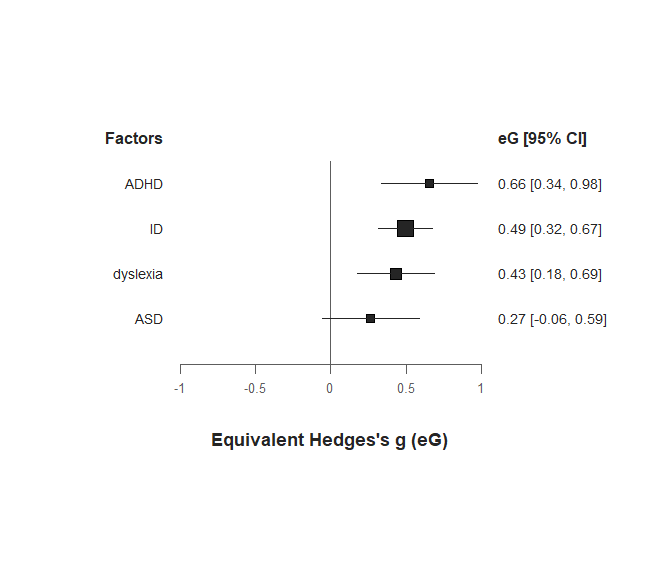The effect sizes are displayed as eG, the default in this function. Since all factors have used OR, we can convert the effect sizes in eOR using the measure argument. Moreover, because all effect sizes are OR and not eOR, we indicate it in the plot (we can do this using the main_value argument to modify the title of the value column and using the main_x_axis argument to modify the title of the x-axis).
In the meantime, we also add a title to the plot using the main_title argument.

forest(umb,
measure = "eOR", # display eOR instead of eG
main_value = "OR [95% CI]", # header of the column displaying the effect sizes
main_x_axis = "Odds Ratio (OR)", # title of the x axis
main_title = "Umbrella review of risk factors for\n neurodevelopmental disorders" # title of the plot
)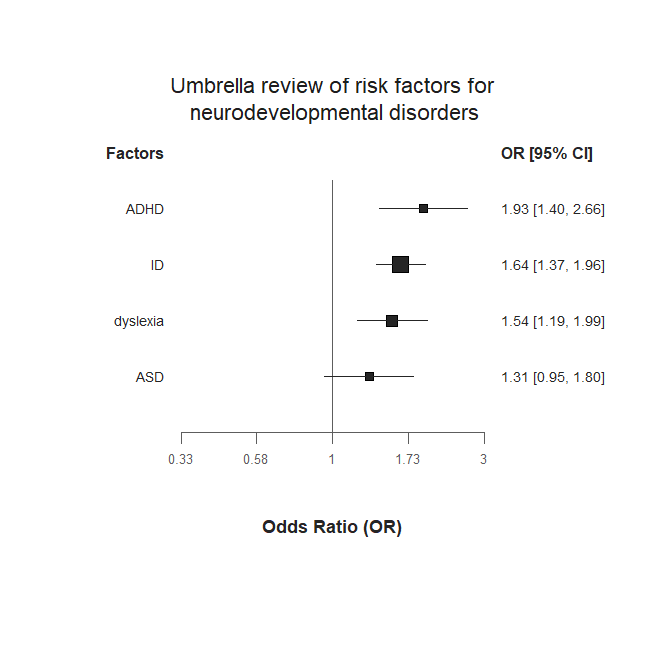As you can see in the code for the title, we have added “backslash” n after the word ‘for’. This forces a line break.
If you find that the title is too large (or too small) compared to the plot, you can modify its size using the cex_title argument. Here, we reduce its size.

forest(umb,
measure = "eOR",
main_value = "OR [95% CI]",
main_x_axis = "Odds Ratio (OR)",
main_title = "Umbrella review of risk factors for\n neurodevelopmental disorders",
cex_title = 1.1, # size of the title, default set as 1.4
)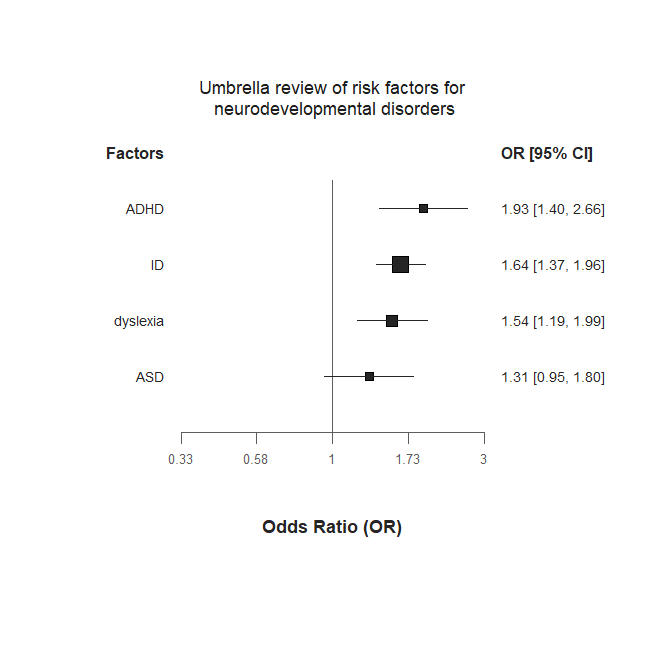The size of the dots reflects the precision of the pooled effect sizes. The narrower the 95% CI, the larger the size of the dot. If you find that the size of the dots is too disproportionate between the factors, you have two options.

1. Rather than using our raw index of precision to scale the size of the dots, you can take its natural logarithm (using the log_cex_dots argument). This will automatically reduces the variability in the size of the dots.
forest(umb,
measure = "eOR",
main_value = "OR [95% CI]",
main_x_axis = "Odds Ratio (OR)",
main_title = "Umbrella review of risk factors for\n neurodevelopmental disorders",
cex_title = 1.1,
log_cex_dots = TRUE # the natural log of the index of precision is used to scale the size of the dots
)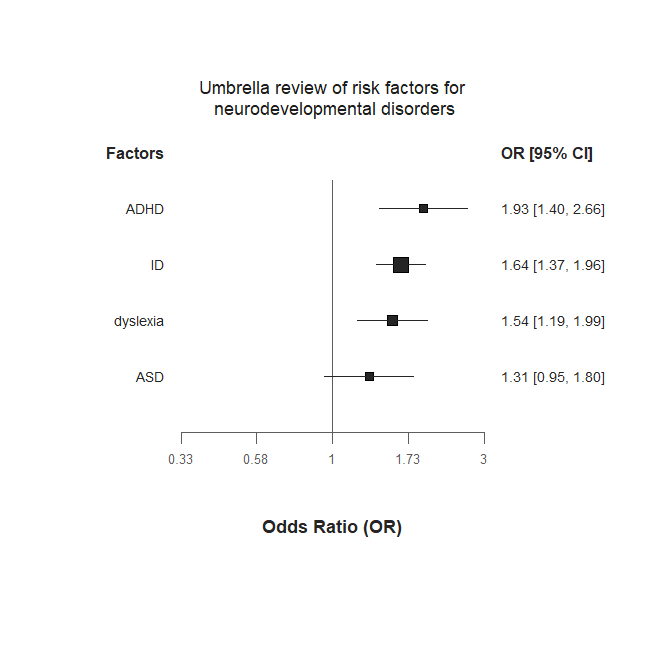1. If the dot representation still does not suit you, you can simply request all the dots to have the same size. The larger the numeric value provided in the fix_size_dots argument, the larger the size of the dots.
forest(umb,
measure = "eOR",
main_value = "OR [95% CI]",
main_x_axis = "Odds Ratio (OR)",
main_title = "Umbrella review of risk factors for\n neurodevelopmental disorders",
cex_title = 1.1,
fix_size_dots = 1.5 # fix the size of the dots
)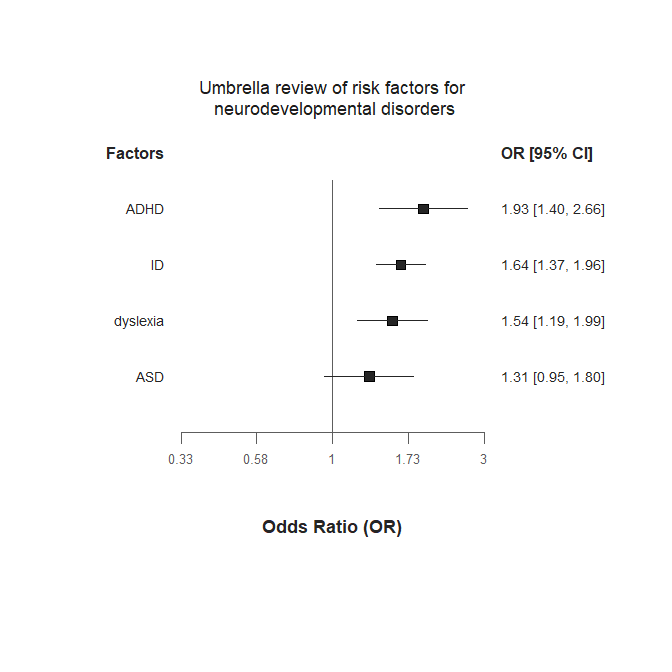# Example 2: modified forest plot (modification of column positions)

This example uses two datasets named df.SMD and df.HR distributed along with the metaumbrella package. For the example, we stratify the evidence for all factors included in these two datasets according to some Personalized criteria.

# perform the calculations
umb <- union.umbrella(umbrella(df.SMD), umbrella(df.HR))

# stratify the evidence
class_I = c(n_studies = 10, total_n = 3000, egger_p = .10, esb_p = .05),
class_II = c(n_studies = 10, total_n = 2000, egger_p = .10),
class_III = c(n_studies = 10, total_n = 1000, egger_p = .10),
class_IV = c(n_studies = 10, total_n = 500, egger_p = .10))

# plot the results
forest(strat.prso)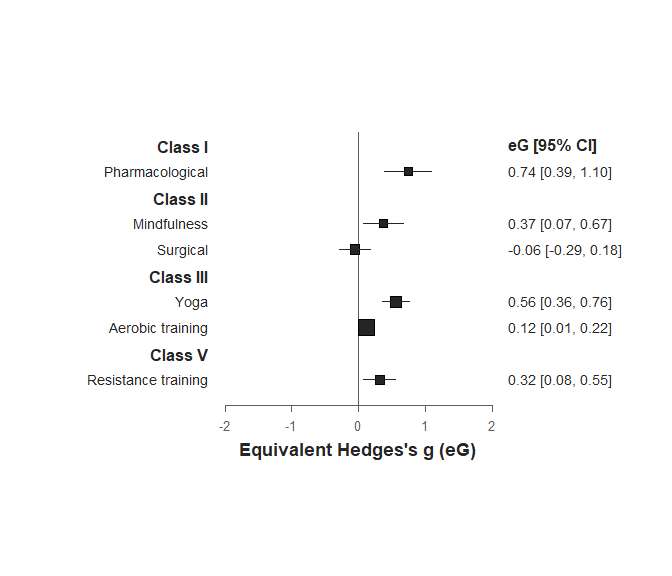As you can see, the results are ordered according to the class reached. The meta-analyses using HR effect size measures have been automatically converted to eG.

In some situations, you may be interested in modifying the position of the value column so that it is presented at the left of the plot. To do so, you have to:
• shift the ‘factor’ column to the left (via the xlim_factor argument) and
• shift the ‘value’ column right to the left of the plot (via the xlim_value argument).

However, before moving the ‘factor’ column to the left, you have first to increase the size of the left area of the plot (via the xlim argument)

forest(strat.prso,
xlim = c(-8, 4), # this increases the size of the left area of the plot
xlim_factor = -6 # this moves the factors column to the left
)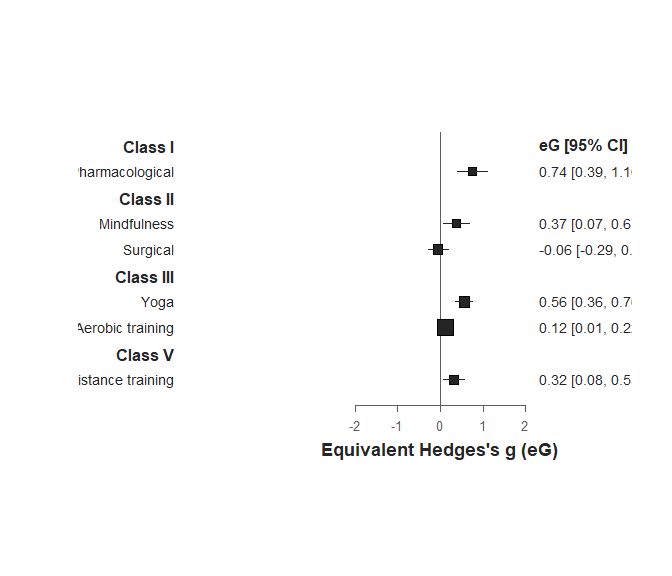Once the ‘factor’ column has been moved, we can move the value column using the xlim_value.

forest(strat.prso,
xlim = c(-8, 4),
xlim_factor = -6,
xlim_value = -3.5 # this moves the value column to the left
)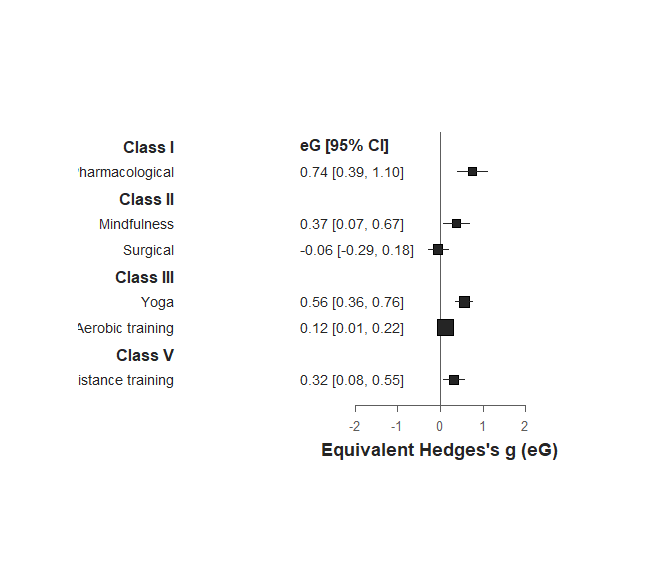Putting the columns side by side makes clear that their centering is different. By default, the factor column in right-aligned while the value column is left-aligned.
Let’s start by changing the alignment of the value column.

forest(strat.prso,
xlim = c(-8, 4),
xlim_factor = -6,
xlim_value = -3.5,
pos_value = "center" # change the alignment of the value column
)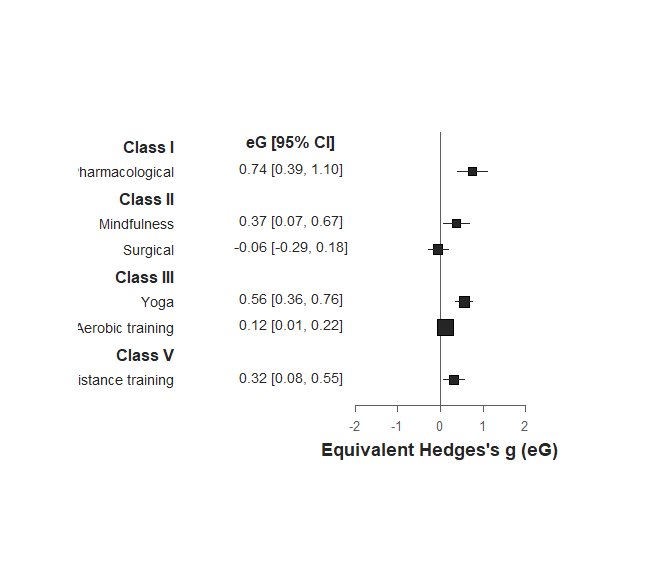Now, we can adjust the alignment of the factor column.

forest(strat.prso,
xlim = c(-8, 4),
xlim_factor = -6,
xlim_value = -3.5,
pos_value = "center",
pos_text = "center" # change the alignment of the factor column
)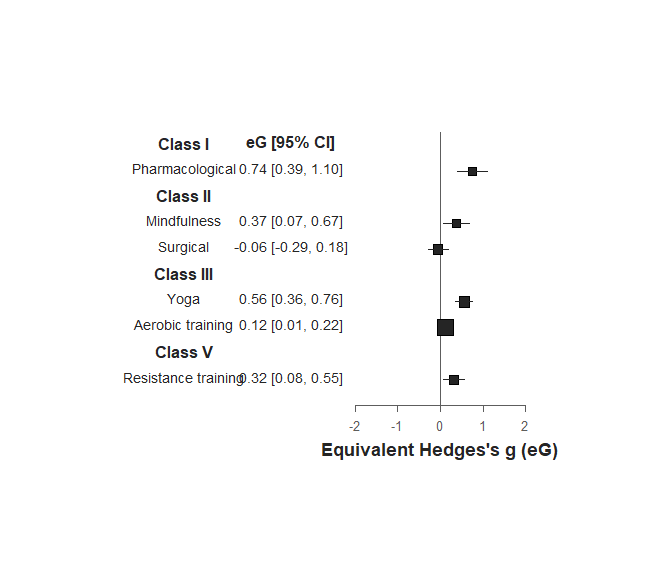The plot is now almost ready. To finish, we can spread slightly the two columns and decrease the overall width of the figure by decreasing the value of the x-limits of the plot using the xlim argument.

forest(strat.prso,
xlim = c(-6.8, 2), # adjust the x-limits of the plot.
xlim_factor = -6,
xlim_value = -3.5,
pos_value = "center",
pos_text = "center"
)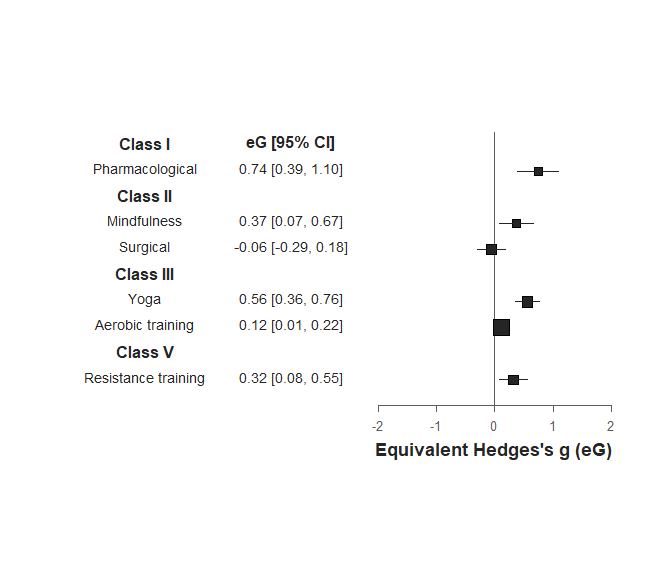# Example 3: modified forest plot (addition of new columns)

This example uses several fictitious datasets distributed along with the metaumbrella package, namely df.SMD, df.OR, df.RR, df.IRR and df.OR.multi. We stratify the evidence for all factors included in these datasets according to the Ioannidis classification

# perform the calculations
umb <- union.umbrella(union.umbrella(
union.umbrella(union.umbrella(
umbrella(df.SMD), umbrella(df.OR)),
umbrella(df.RR)), umbrella(df.IRR)),
umbrella(df.OR.multi, mult.level = TRUE))

strat.prso <- add.evidence(umb, criteria = "Ioannidis")

forest(strat.prso)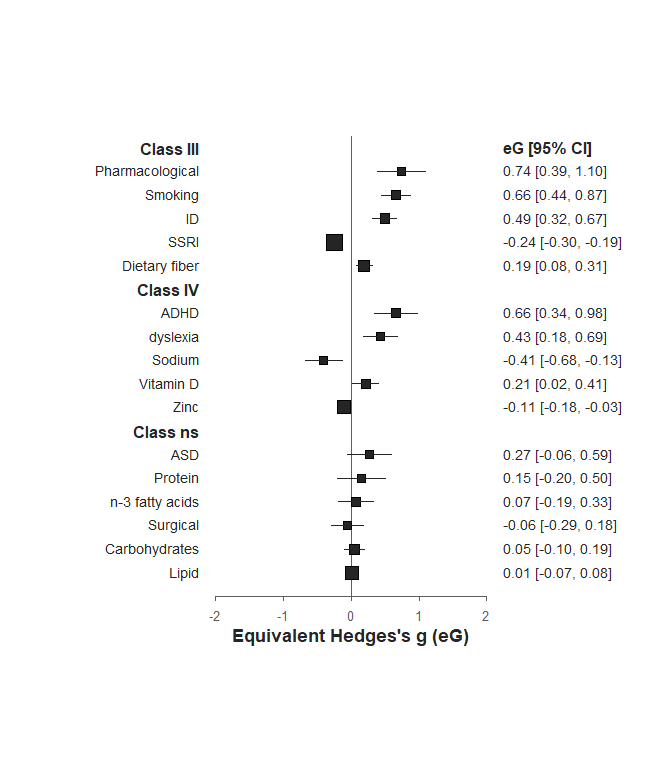In this example, we will see how to display more information on the meta-analyses included in the umbrella review. Let’s imagine that you are interested in plotting the name of the first author of the original meta-analyses (stored in the meta-review column of the original datasets) and the number of cases.

First, we need to extract the name of the first author of the meta-analyses in the original dataset.

list_authors <- lapply(list(df.SMD, df.OR, df.RR, df.IRR, df.HR, df.OR.multi),
function(x) cbind(author = unique(x$meta_review), factor = unique(x$factor)))

authors <- do.call(rbind, list_authors)
authors
##       author       factor
##  [1,] "Smith"      "Pharmacological"
##  [2,] "Smith"      "Surgical"
##  [4,] "Garcia"     "ASD"
##  [5,] "Garcia"     "dyslexia"
##  [6,] "Garcia"     "ID"
##  [7,] "el-Hakim"   "SSRI"
##  [8,] "Pham"       "Smoking"
##  [9,] "al-Ishak"   "Yoga"
## [10,] "al-Ishak"   "Aerobic training"
## [11,] "al-Ishak"   "Resistance training"
## [12,] "al-Ishak"   "Mindfulness"
## [13,] "el-Can"     "n-3 fatty acids"
## [14,] "Bown"       "Protein"
## [15,] "el-Abood"   "Carbohydrates"
## [16,] "Hinostroza" "Lipid"
## [17,] "al-Rasul"   "Dietary fiber"
## [18,] "Ortega"     "Sodium"
## [19,] "Nelson"     "Zinc"
## [20,] "Huynh"      "Vitamin D"

We now have the list of first authors of the original meta-analyses associated with the different factors. However, in the plot, the factors are ranked according to their class obtained in the stratification of evidence. Therefore, they are not in the same order as the information extracted. To reorganize this dataset, we need to use the information on the order of the factors which is automatically returned by the forest function.

order_factors_plot <- forest(strat.prso)

# this allows to obtain the order of the factors in the plot
order_factors <- order_factors_plot$factor$factor

# now that we have the correct order, we simply have to reorganize the dataset according to this information
authors_ordered <- authors[match(order_factors, authors[, "factor"]), ]
authors_ordered
##   "Pharmacological" "Smoking"         "ID"              "SSRI"
##   "Dietary fiber"   "ADHD"            "dyslexia"        "Sodium"
##   "Vitamin D"       "Zinc"            "ASD"             "Protein"
##  "n-3 fatty acids" "Surgical"        "Carbohydrates"   "Lipid"
##       author       factor
##  [1,] "Smith"      "Pharmacological"
##  [2,] "Pham"       "Smoking"
##  [3,] "Garcia"     "ID"
##  [4,] "el-Hakim"   "SSRI"
##  [5,] "al-Rasul"   "Dietary fiber"
##  [7,] "Garcia"     "dyslexia"
##  [8,] "Ortega"     "Sodium"
##  [9,] "Huynh"      "Vitamin D"
## [10,] "Nelson"     "Zinc"
## [11,] "Garcia"     "ASD"
## [12,] "Bown"       "Protein"
## [13,] "el-Can"     "n-3 fatty acids"
## [14,] "Smith"      "Surgical"
## [15,] "el-Abood"   "Carbohydrates"
## [16,] "Hinostroza" "Lipid"
The authors_ordered object now stores the name of the authors in the same order as in the plot. We simply have to add the information to the plot.
• The add_columns argument allows to pass a vector (or a dataframe) which will be added to the plot.
• The main_add_columns argument allows to specify the names of the new columns (by default, the names of the columns are those used in the input object).
• The xlim_add_columns argument allows to specify the position of the new columns on the x-axis.

We will add these new columns on the left side of the plot. As in the previous example, because we include more information on the left side of the plot, we need to increase the width of the plot on the left area.

# we create the variable displaying the number of studies and total sample size
sum <- summary(strat.prso)

# as you can see, the order of the summary function and of the forest function is identical. We do not need to reorder this information.
stud_total_n = paste0(sum\$n_cases)

forest(strat.prso,
add_columns = data.frame(authors_ordered[, "author"], stud_total_n), # new columns
main_add_columns = c("Authors", "Studies\n(sample size)"), # name of the new columns
xlim_add_columns = c(-7.5, -5), # position of the new columns
xlim = c(-10, 4.5) # increase the width of the plot on the left side
)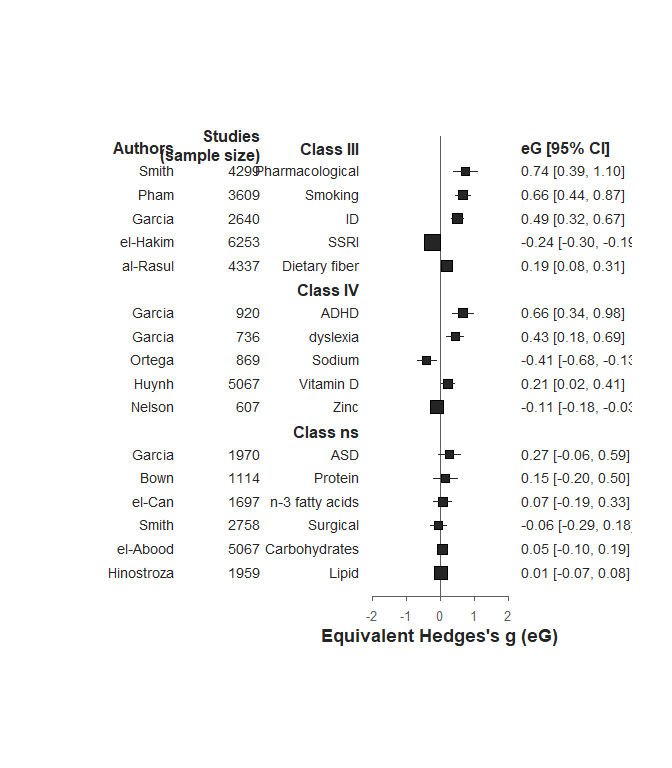Not too bad. We will now adjust the centering of the columns on the left side of the plot using the pos_text argument.

forest(strat.prso,
xlim = c(-10, 5),
pos_text = "center" # center the factors columns and the two columns added to the plot
)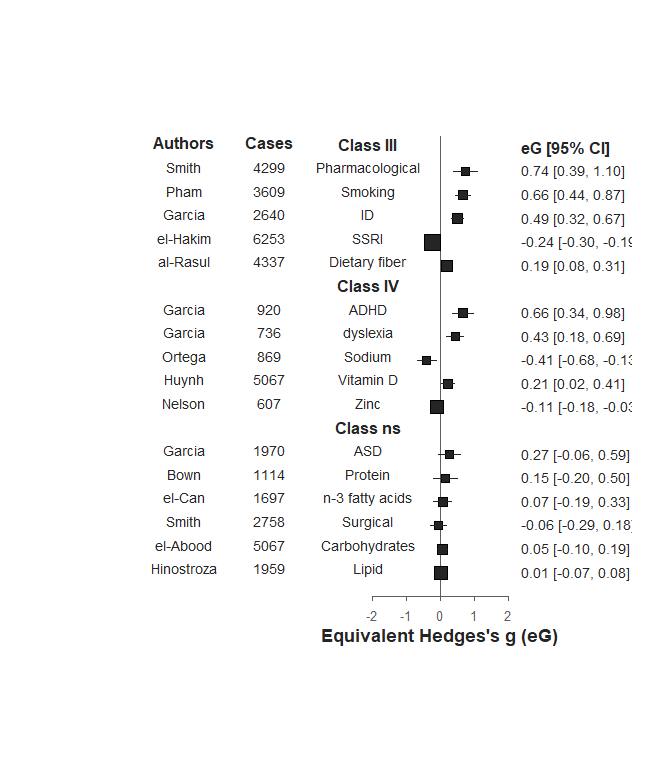The columns are now a bit too close to the plot. We will adjust their x-axis positions.

forest(strat.prso,
xlim_add_columns = c(-9.5, -7), # adjust the x-axis position of the new columns
xlim = c(-10, 5),
pos_text = "center",
xlim_factor = -4 # adjust the x-axis position of the factor column
)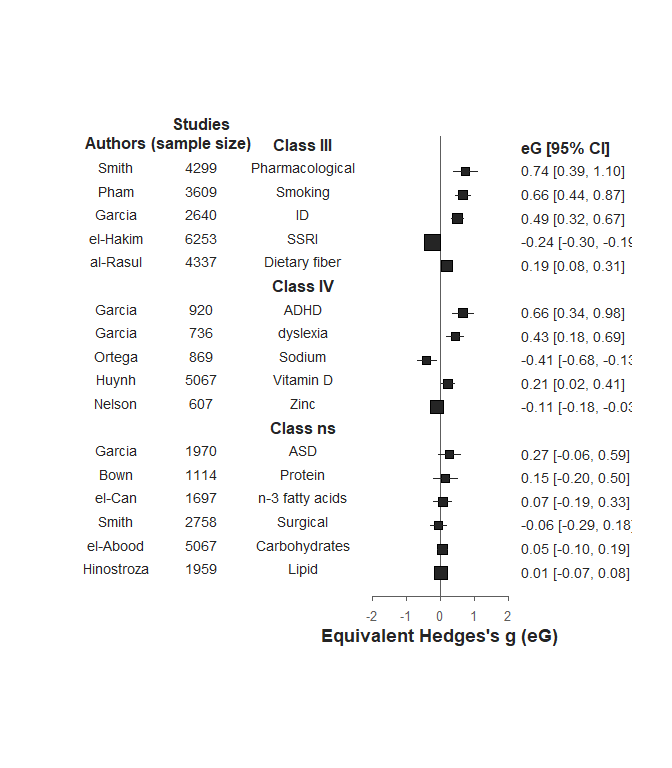If you find that the number of meta-analyses displayed is too high, you can restrict to some classes. For example, you can be interested in presenting factors reaching at least a class IV. This can be done using the print.classes argument.

forest(strat.prso,
xlim = c(-10, 5),
pos_text = "center",
xlim_factor = -4,
print.classes = c("I", "II", "III", "IV") # request to print only classes I to IV

)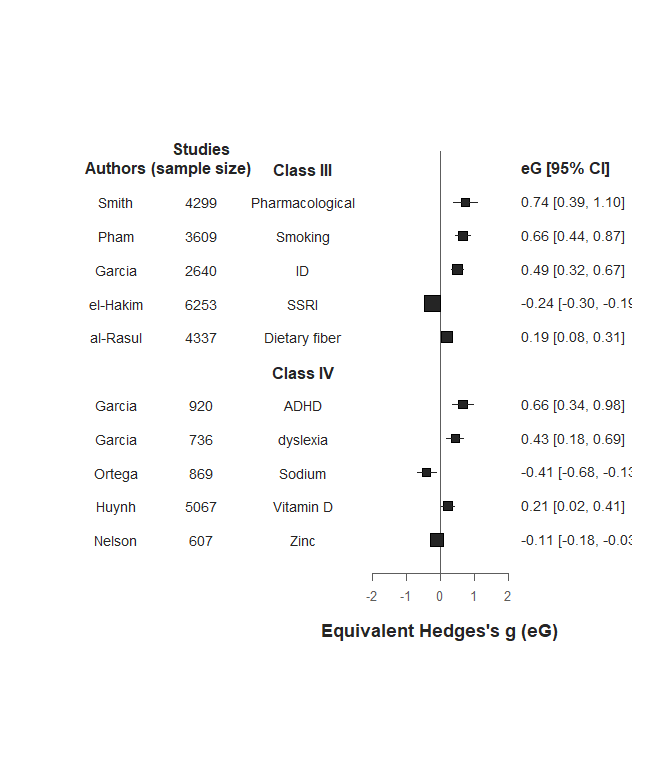Finally, if you find that the text in the columns and the dots are too large compared to the plot, you can reduce their size.

forest(strat.prso,
)